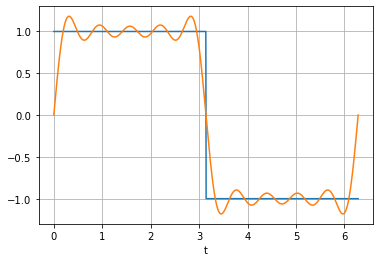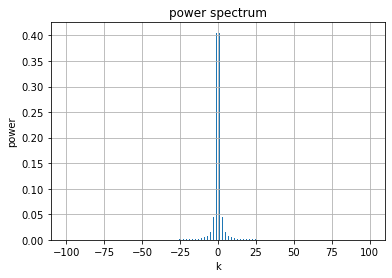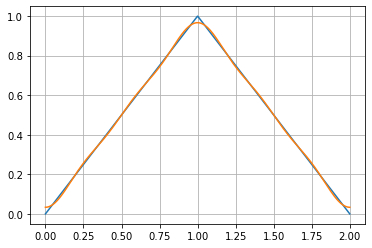In :
from pylab import *


## Python ranges and conditionals¶

In :
range(1, 3)   # like matlab [1:2]
range(1, 5+1, 2)   # like matlab [1:2:5]

Out:
range(1, 6, 2)
In :
a = 2
if a > 3:
print('hello')
elif a == 2:
pass
else:
print('stuff')


## Computer approximations $p_n$¶

In :
t = linspace(0, 2*pi, 1000)
a0 = 0
approx = a0*1
n = 10
# note to get n, you need to use range k+1
for k in range(1, n+1, 2):
approx = approx + (4/pi)*sin(k*t)/k

In :
f = where(t > pi, -1, 1)

plot(t, f)
plot(t, approx)
grid()
xlabel('t')

Out:
Text(0.5, 0, 't')## Power Spectrum¶

In :
ak_list = []
n = 100
k_list = range(-n, n+1)

for k in k_list:
if k == 0:
ak_list.append(0)
elif k % 2 == 0:  #even
ak_list.append(0)
else:  # odd and non-zero
ak_list.append(2*1j/(pi*k))

# turn list into
ak_list = np.array(ak_list)

In :
power = abs(ak_list)**2
bar(k_list, power)
xlabel('k')
ylabel('power')
title('power spectrum')
grid()## Computing || f - p_n ||^2¶

In :
from pylab import *

norm_f_sq = 1

norm_error_sq = norm_f_sq
n = 100
for k in range(-n, n+1):  # always range uses n+1
if k == 0: # a0
ak = 0
elif k%2 != 0: # odd terms
ak = 2*1j/(pi*k)
elif k%2 == 0: # even
ak = 0
# same as: norm_error_sq = norm_error_sq - abs(ak)**2
norm_error_sq -= abs(ak)**2

print('should converge to zero as n approaches infinity')
norm_error_sq

should converge to zero as n approaches infinity

Out:
0.004052712269689609

# Example from Class 2/12/21¶

In :
norm_f_sq = 1/3

norm_error_sq = norm_f_sq
n  = 5
for k in range(-n, n+1):
if k == 0:
ak = 1/2
elif k %2 == 0:
ak = 0
else:
ak = -2/(k**2*pi**2)
norm_error_sq -= abs(ak)**2

norm_error_sq

Out:
6.014654969650223e-05
In :
error_sq = 1/3 - 1/4  # ||f||^2 - |a0|^2
for k in range(-n, n+1):
if k != 0 and k % 2 == 1:  # k non zeros and k odd
error_sq -= (4/(k**4*pi**4))  # |ak|^2
error_sq

Out:
6.014654969650212e-05
In :
t = linspace(0, 2, 1000)
f = where(t < 1, t, 2 - t)
plot(t, f)

pn = 1/2

for k in range(1, n+1, 2):
pn -= (4/(pi**2))*cos(k*pi*t)/k**2

plot(t, pn)
grid()In [ ]:
a9